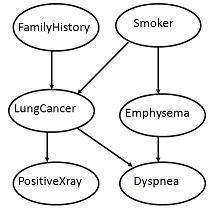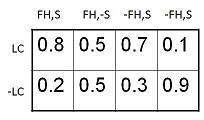# Data Mining - Bayesian Classification

Bayesian classification is based on Bayes' Theorem. Bayesian classifiers are the statistical classifiers. Bayesian classifiers can predict class membership probabilities such as the probability that a given tuple belongs to a particular class.

## Baye's Theorem

Bayes' Theorem is named after Thomas Bayes. There are two types of probabilities −

• Posterior Probability [P(H/X)]
• Prior Probability [P(H)]

where X is data tuple and H is some hypothesis.

According to Bayes' Theorem,

P(H/X)= P(X/H)P(H) / P(X)

## Bayesian Belief Network

Bayesian Belief Networks specify joint conditional probability distributions. They are also known as Belief Networks, Bayesian Networks, or Probabilistic Networks.

• A Belief Network allows class conditional independencies to be defined between subsets of variables.

• It provides a graphical model of causal relationship on which learning can be performed.

• We can use a trained Bayesian Network for classification.

There are two components that define a Bayesian Belief Network −

• Directed acyclic graph
• A set of conditional probability tables

## Directed Acyclic Graph

• Each node in a directed acyclic graph represents a random variable.
• These variable may be discrete or continuous valued.
• These variables may correspond to the actual attribute given in the data.

## Directed Acyclic Graph Representation

The following diagram shows a directed acyclic graph for six Boolean variables.The arc in the diagram allows representation of causal knowledge. For example, lung cancer is influenced by a person's family history of lung cancer, as well as whether or not the person is a smoker. It is worth noting that the variable PositiveXray is independent of whether the patient has a family history of lung cancer or that the patient is a smoker, given that we know the patient has lung cancer.

## Conditional Probability Table

The conditional probability table for the values of the variable LungCancer (LC) showing each possible combination of the values of its parent nodes, FamilyHistory (FH), and Smoker (S) is as follows −